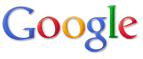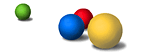A   A B C D E F G H J K L M N P Q S T W X Y Z
 B   A B C D E F G H J K L M N P Q S T W X Y Z
 C   A B C D E F G H J K L M N P Q S T W X Y Z
 D   A B C D E F G H J K L M N P Q S T W X Y Z
 E   A B C D E F G H J K L M N P Q S T W X Y Z
 F   A B C D E F G H J K L M N P Q S T W X Y Z
 G   A B C D E F G H J K L M N P Q S T W X Y Z
 H   A B C D E F G H J K L M N P Q S T W X Y Z
 J   A B C D E F G H J K L M N P Q S T W X Y Z
 K   A B C D E F G H J K L M N P Q S T W X Y Z
 L   A B C D E F G H J K L M N P Q S T W X Y Z
 M   A B C D E F G H J K L M N P Q S T W X Y Z
 N   A B C D E F G H J K L M N P Q S T W X Y Z
 P   A B C D E F G H J K L M N P Q S T W X Y Z
 Q   A B C D E F G H J K L M N P Q S T W X Y Z
 S   A B C D E F G H J K L M N P Q S T W X Y Z
 T   A B C D E F G H J K L M N P Q S T W X Y Z
 W   A B C D E F G H J K L M N P Q S T W X Y Z
 X   A B C D E F G H J K L M N P Q S T W X Y Z
 Y   A B C D E F G H J K L M N P Q S T W X Y Z
 Z   A B C D E F G H J K L M N P Q S T W X Y Z